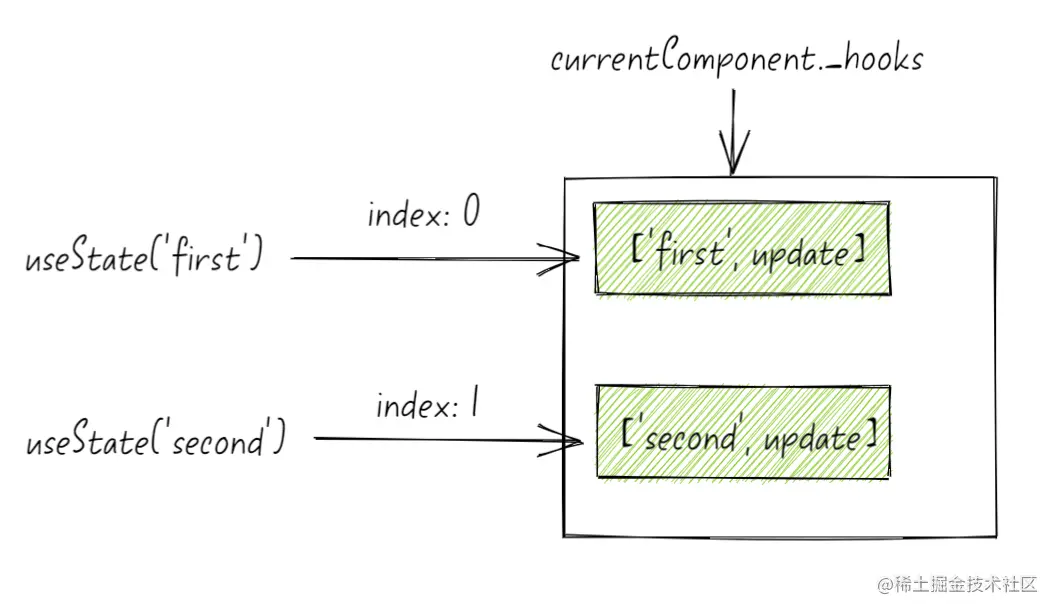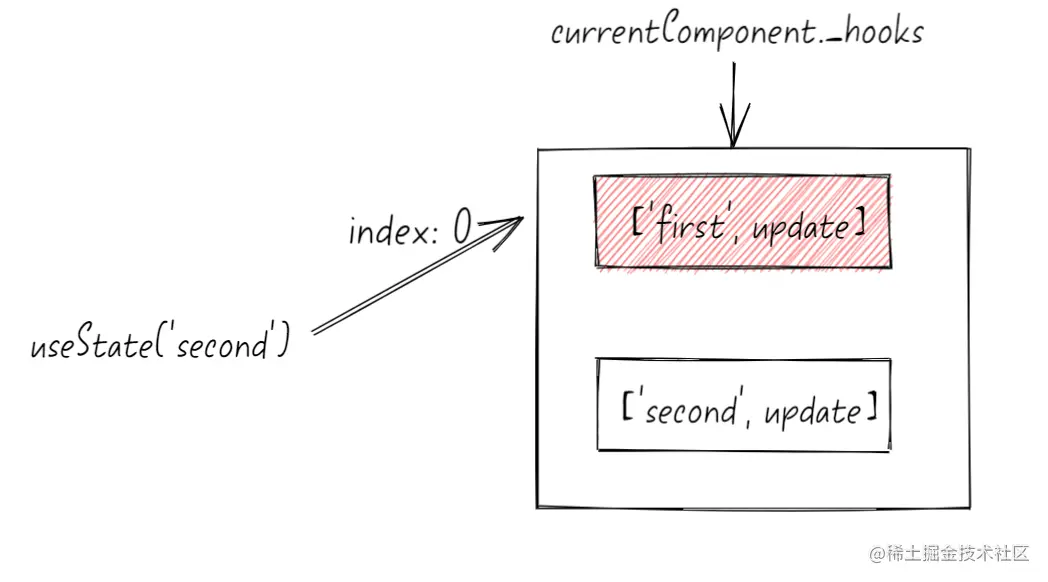# 我打破了 React Hook 必须按顺序、不能在条件语句中调用的枷锁React 官网介绍了 Hook 的这样一个限制：

``````function App(){
if (xxx) {
return null;
}

// ❌ React Hook "useState" is called conditionally.
// React Hooks must be called in the exact same order in every component render.
useState();

return 'Hello'
}
``````

## 限制的原因

``````// 当前正在运行的组件
let currentComponent

// 当前 hook 的全局索引
let currentIndex

// 第一次调用 currentIndex 为 0
useState('first')

// 第二次调用 currentIndex 为 1
useState('second')
``````

``````// 当前正在运行的组件
let currentComponent

// 当前 hook 的全局索引
let currentIndex

// 第一次调用 currentIndex 为 0
if (Math.random() > 0.5) {
useState('first')
}

// 第二次调用 currentIndex 为 1
useState('second')
``````

``````_hooks: [
{ value: 'first', update: function },
{ value: 'second', update: function },
]
````````````export default function App() {
if (Math.random() > 0.5) {
useState(10000)
}
const [value, setValue] = useState(0)

return (
<div>
<button onClick={() => setValue(value + 1)}>+</button>
{value}
</div>
)
}
``````## 破解限制

``````export default function App() {
if (Math.random() > 0.5) {
useState(10000, 'key1');
}
const [value, setValue] = useState(0, "key2");

return (
<div>
<button onClick={() => setValue(value + 1)}>+</button>
{value}
</div>
);
}
``````

``````_hooks: [
{ value: 'first', update: function },
{ value: 'second', update: function },
]
``````

``````_hooks: [
key1: { value: 'first', update: function },
key2: { value: 'second', update: function },
]
``````

## 改造源码

``````export function useState(initialState) {
currentHook = 1;
return useReducer(invokeOrReturn, initialState, undefined);
}
``````

``````+ export function useState(initialState, key) {
currentHook = 1;
+ return useReducer(invokeOrReturn, initialState, undefined, key);
}
``````

`useReducer` 原本是通过全局索引去获取 Hook state：

``````// 全局索引
let currentIndex

export function useReducer(reducer, initialState, init) {
const hookState = getHookState(currentIndex++, 2);
hookState._reducer = reducer;

return hookState._value;
}
``````

``````// 全局索引
let currentIndex

+ export function useReducer(reducer, initialState, init, key) {
+  const hookState = getHookState(key || currentIndex++, 2);
hookState._reducer = reducer;

return hookState._value;
}
``````

``````function getHookState(index, type) {
const hooks =
currentComponent.__hooks ||
(currentComponent.__hooks = {
_list: [],
_pendingEffects: [],
});

// 传入 key 值是 string 或 symbol 都可以
+  if (typeof index !== 'number') {
+    if (!hooks._list[index]) {
+      hooks._list[index] = {};
+    }
+  } else {
if (index >= hooks._list.length) {
hooks._list.push({});
}
}
// 这里天然支持 key 值取用的方式
return hooks._list[index];
}
``````

``````export default function App() {
if (Math.random() > 0.5) {
useState(10000, 'key1');
}
const [value, setValue] = useState(0, 'key2');

return (
<div>
<button onClick={() => setValue(value + 1)}>+</button>
{value}
</div>
);
}
``````## 自动编译

``````const valueKey = Symbol();

function useFormInput() {
const [value, setValue] = useState(valueKey);
return {
value,
onChange(e) {
setValue(e.target.value);
},
};
}
``````

``````function Form() {
// 使用 Symbol
const name = useFormInput();
// 又一次使用了同一个 Symbol
const surname = useFormInput();
// ...
return (
<>
<input {...name} />
<input {...surname} />
{/* ... */}
</>
)
}
``````

``````traverse(node) {
if (isReactHookInvoking(node)) {
}
}
``````

``````function Form() {
+  const name = useFormInput('key_1');
+  const surname = useFormInput('key_2');
// ...
return (
<>
<input {...name} />
<input {...surname} />
{/* ... */}
</>
)
}

+ function useFormInput(key) {
+  const [value, setValue] = useState(key);
return {
value,
onChange(e) {
setValue(e.target.value);
},
};
}
``````

key 的生成策略可以是随机值，也可以是注入一个 Symbol，这个无所谓，保证运行时期不会改变即可。也许有一些我没有考虑周到的地方，对此有任何想法的同学都欢迎加我微信 sshsunlight 讨论，当然单纯的交个朋友也没问题，大佬或者萌新都欢迎。

## 总结

• 介绍 Hook 实现的大概原理以及限制
• 探索出修改源码机制绕过限制的方法